# High School Physics : Understanding Heat and Work

## Example Questions

### Example Question #1 : Understanding Heat And Work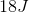of heat is added to a gas in a closed container. If the lid to the container is lifted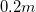with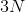of force, what is the net energy added to the system?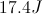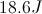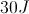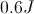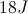Explanation:

The formula for the net energy added to a system is;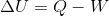The change in energy equals the heat energy minus the work done.

We don't know the work, but we can solve for it using the work equation: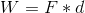We are given both the force and the distance, allowing us to calculate the work.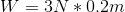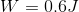Now that we know both the work done and the heat added, we can solve for the final energy of the system.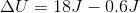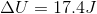### Example Question #2 : Understanding Heat And Work

A certain amount of heat energy is added to a closed system. A few moments later, a scientist observes that the total increase in energy is LESS than that heat energy added to the system. Which could be a valid explanation for this conclusion?

External forces are acting upon the system

The system is not actually closed

The measuring tools were incorrectly calibrated

The system is imperfectly designed

Work was done by the system

Work was done by the system

Explanation:

The most likely explanation is that work is done by the system.

The formula for change in energy shows that the net change in energy is equal to the increase in heat energy minus the work done:Since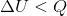, there must have been work done by the system.

### Example Question #3 : Understanding Heat And Work

A bathtub and a coffee cup both contain water at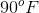. Which of the following is true?

The bathtub contains more heat, and the two have the same temperature

The two contain the same amount of heat, and the bathtub has a higher temperature

The cup contains more heat, and the two have the same temperature

The two contain the same amount of heat and have the same temperature

The bathtub contains more heat, and the two have the same temperature

Explanation:

Heat is a form of energy, while temperature is a measure of the average kinetic energy of the molecules present in a system. Since both systems are measure to be at, their average kinetic energies are the same. The cup and the bathtub have the same temperature; however, since the bathtub contains more water, it contains more molecules. Temperature is the measure of heat energy per molecule. A greater number of molecules at the same temperature is indicative of more heat energy than fewer molecules at that temperature. Since the bathtub has more molecules, it has more heat energy even though the two systems have the same temperature.

### Example Question #4 : Understanding Heat And Work

In order for heat transfer to occur, which of the following must be present?

Interface between two mediums

Air

All of these must be present for heat transfer to occur